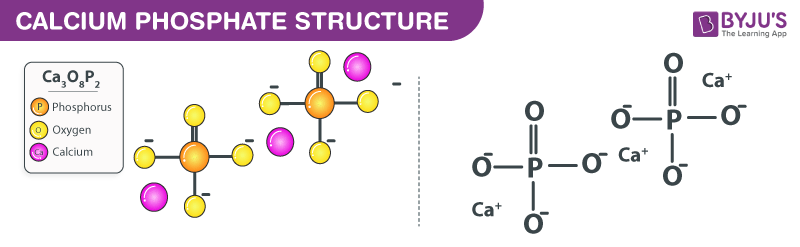# Calcium Phosphate Formula

Knowing about the calcium phosphate formula can be helpful for students. The chemical compound is found relatively in many living organisms. Calcium phosphate is a chemical compound that mainly contains calcium ions along with inorganic phosphate anions. The mineral is typically an odourless solid which is white in colour. It is also highly soluble in water. Additionally, calcium phosphate minerals are used in a wide variety of applications including production of phosphoric acid and fertilizers.

## Calcium Phosphate Chemical Formula

Calcium phosphate chemical formula is given as Ca3(PO4)2. The formula arises as calcium phosphate is generally composed of two atoms of phosphorus, three atoms of calcium and eight oxygen atoms.

The ions present in calcium phosphate are the Ca2+ calcium ion and phosphate ion. Calcium phosphate may also be found in combinations of the calcium ion with orthophosphate, pyrophosphate or metaphosphate ions. Due to this the formula might change where monocalcium phosphate’s chemical formula is Ca(H2PO4)2, dicalcium phosphate is given as Ca2HPO4 and tricalcium phosphate formula is represented as Ca3(PO4)2.

 Formula Ca3(PO4)2 Molar Mass 310.174 g/mol Density 3.14 g/cm³ Melting Point 1670 °C Boiling Point Usually decomposes

### Calcium Phosphate Structural Formula

Here is a structural formula of calcium phosphate.